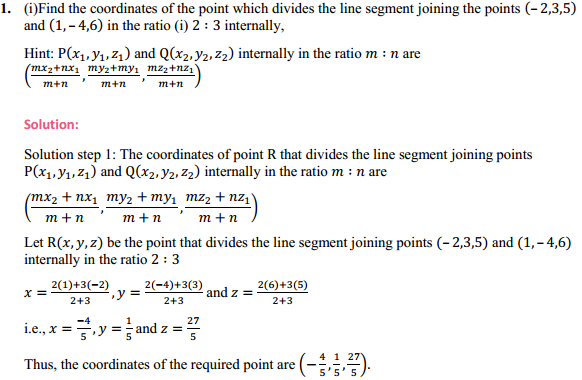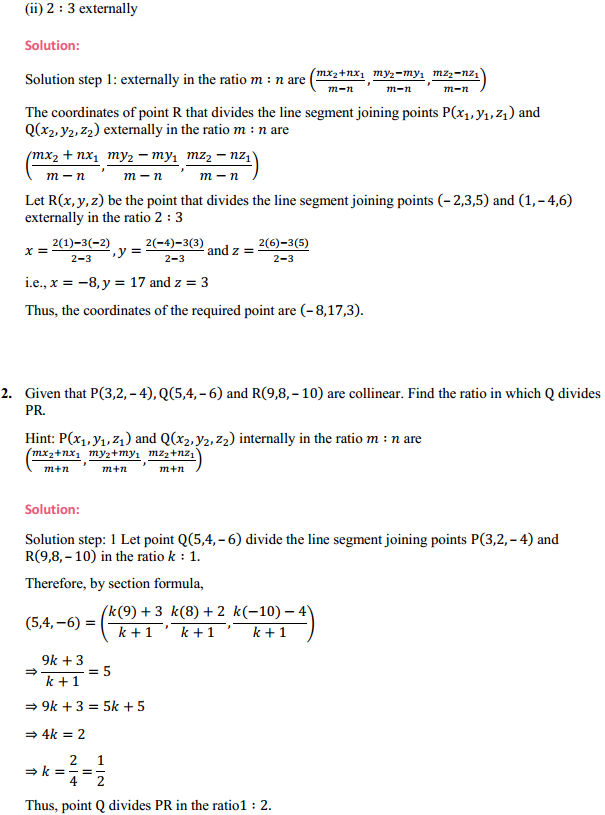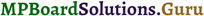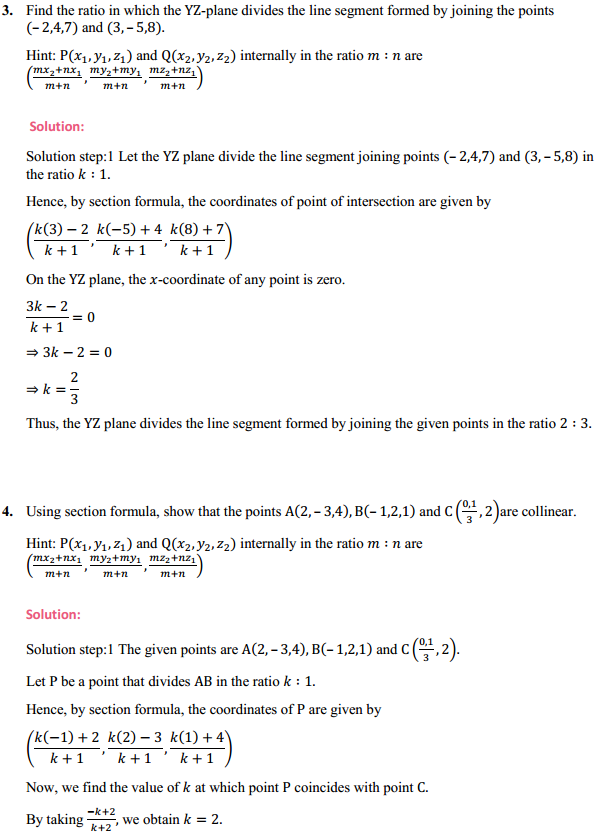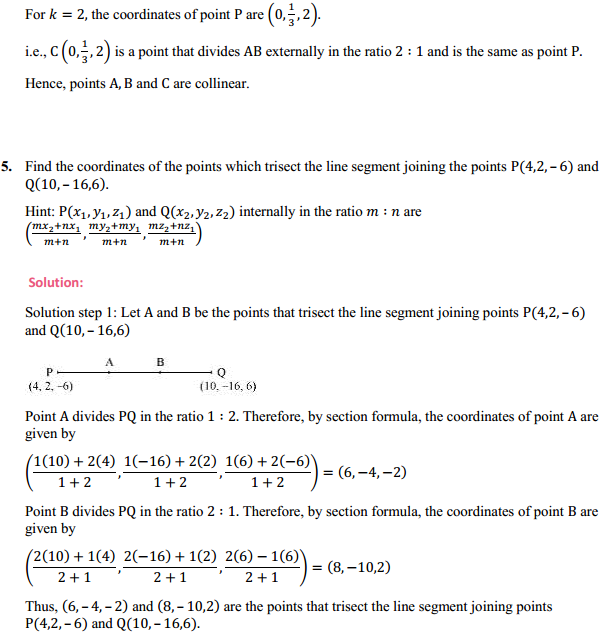# MP Board Class 11th Maths Solutions Chapter 12 Introduction to three Dimensional Geometry Ex 12.3

In this article, we share MP Board Class 11th Maths Book Solutions Chapter 12 Introduction to three Dimensional Geometry Ex 12.3.

## MP Board Class 11th Maths Solutions Chapter 9 Introduction to three Dimensional Geometry Exercise 12.3